Publicité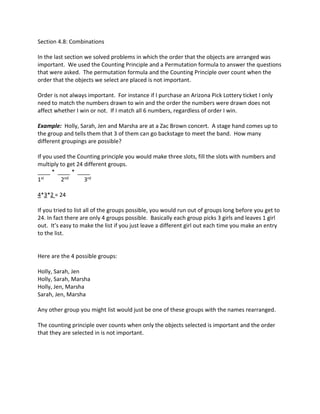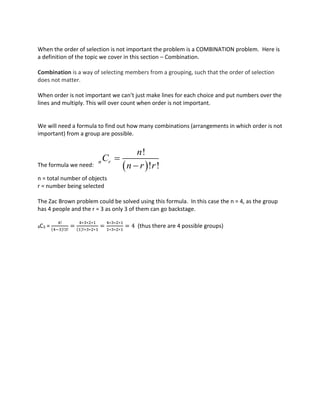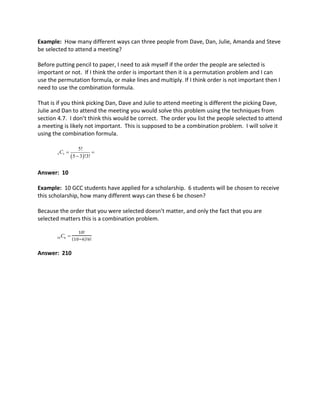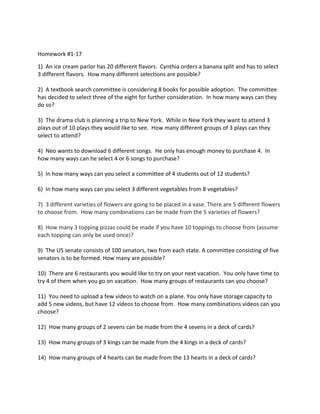Publicité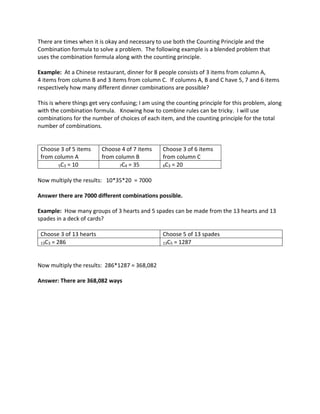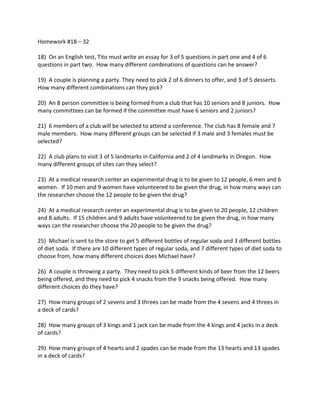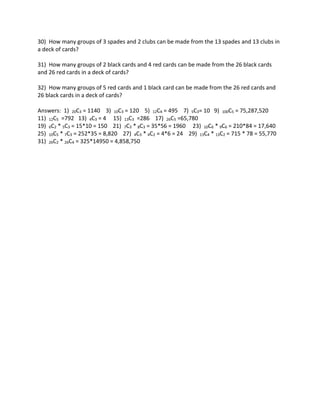Prochain SlideShare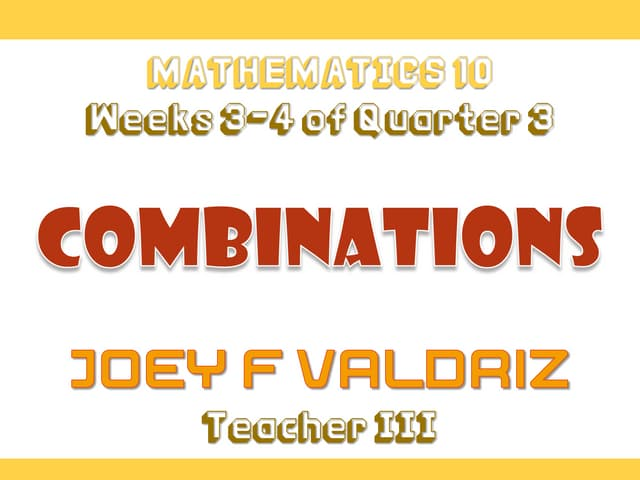Combination
Chargement dans ... 3
1 sur 8
Publicité

### COMBINATION PROBLEMS.pdf

1. Section 4.8: Combinations In the last section we solved problems in which the order that the objects are arranged was important. We used the Counting Principle and a Permutation formula to answer the questions that were asked. The permutation formula and the Counting Principle over count when the order that the objects we select are placed is not important. Order is not always important. For instance if I purchase an Arizona Pick Lottery ticket I only need to match the numbers drawn to win and the order the numbers were drawn does not affect whether I win or not. If I match all 6 numbers, regardless of order I win. Example: Holly, Sarah, Jen and Marsha are at a Zac Brown concert. A stage hand comes up to the group and tells them that 3 of them can go backstage to meet the band. How many different groupings are possible? If you used the Counting principle you would make three slots, fill the slots with numbers and multiply to get 24 different groups. ____ * ____ * ____ 1st 2nd 3rd 4*3*2 = 24 If you tried to list all of the groups possible, you would run out of groups long before you get to 24. In fact there are only 4 groups possible. Basically each group picks 3 girls and leaves 1 girl out. It’s easy to make the list if you just leave a different girl out each time you make an entry to the list. Here are the 4 possible groups: Holly, Sarah, Jen Holly, Sarah, Marsha Holly, Jen, Marsha Sarah, Jen, Marsha Any other group you might list would just be one of these groups with the names rearranged. The counting principle over counts when only the objects selected is important and the order that they are selected in is not important.
2. When the order of selection is not important the problem is a COMBINATION problem. Here is a definition of the topic we cover in this section – Combination. Combination is a way of selecting members from a grouping, such that the order of selection does not matter. When order is not important we can’t just make lines for each choice and put numbers over the lines and multiply. This will over count when order is not important. We will need a formula to find out how many combinations (arrangements in which order is not important) from a group are possible. The formula we need:   ! ! ! n r n C n r r   n = total number of objects r = number being selected The Zac Brown problem could be solved using this formula. In this case the n = 4, as the group has 4 people and the r = 3 as only 3 of them can go backstage. 4C3 = 4! (4−3)!3! = 4∗3∗2∗1 (1)!∗3∗2∗1 = 4∗3∗2∗1 1∗3∗2∗1 = 4 (thus there are 4 possible groups)
3. Example: How many different ways can three people from Dave, Dan, Julie, Amanda and Steve be selected to attend a meeting? Before putting pencil to paper, I need to ask myself if the order the people are selected is important or not. If I think the order is important then it is a permutation problem and I can use the permutation formula, or make lines and multiply. If I think order is not important then I need to use the combination formula. That is if you think picking Dan, Dave and Julie to attend meeting is different the picking Dave, Julie and Dan to attend the meeting you would solve this problem using the techniques from section 4.7. I don’t think this would be correct. The order you list the people selected to attend a meeting is likely not important. This is supposed to be a combination problem. I will solve it using the combination formula.   5 3 5! 5 3 !3! C    Answer: 10 Example: 10 GCC students have applied for a scholarship. 6 students will be chosen to receive this scholarship, how many different ways can these 6 be chosen? Because the order that you were selected doesn’t matter, and only the fact that you are selected matters this is a combination problem. 10 6 C  10! (10−6)!6! Answer: 210